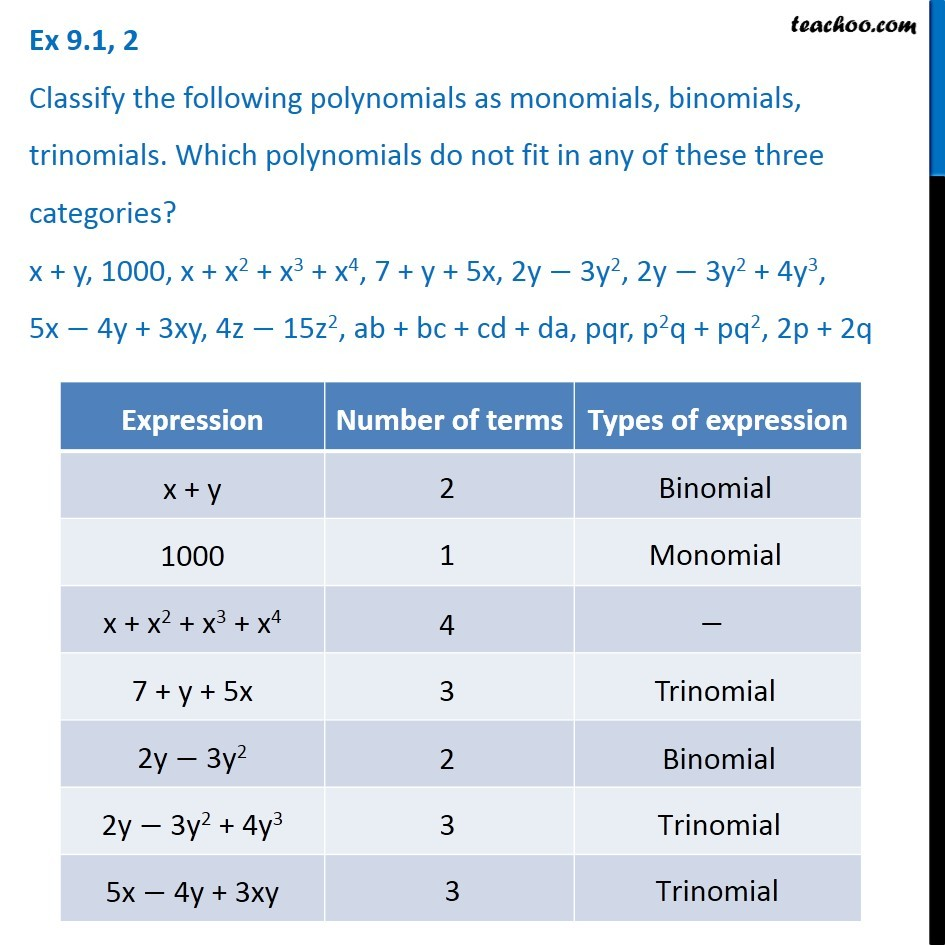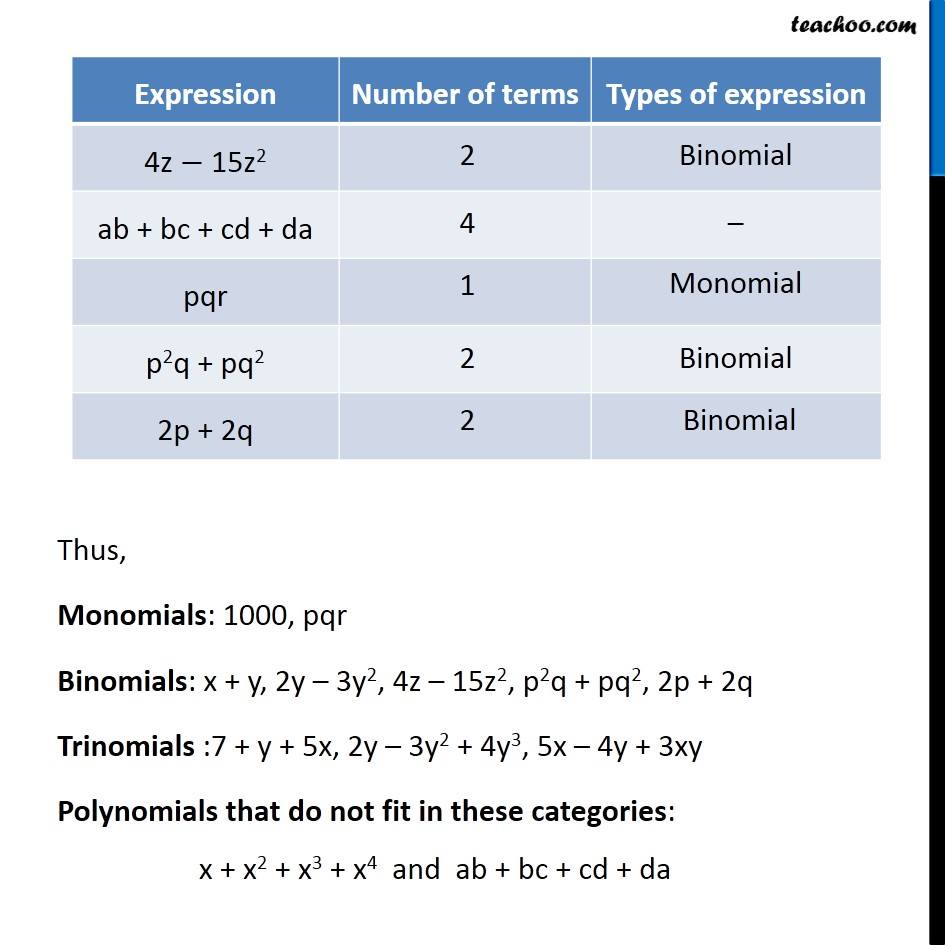Ex 8.1

Chapter 8 Class 8 Algebraic Expressions and Identities
Serial order wiseLearn in your speed, with individual attention - Teachoo Maths 1-on-1 Class

### Transcript

Question 2 Classify the following polynomials as monomials, binomials, trinomials. Which polynomials do not fit in any of these three categories? x + y, 1000, x + x2 + x3 + x4, 7 + y + 5x, 2y − 3y2, 2y − 3y2 + 4y3, 5x − 4y + 3xy, 4z − 15z2, ab + bc + cd + da, pqr, p2q + pq2, 2p + 2q Thus, Monomials: 1000, pqr Binomials: x + y, 2y – 3y2, 4z – 15z2, p2q + pq2, 2p + 2q Trinomials :7 + y + 5x, 2y – 3y2 + 4y3, 5x – 4y + 3xy Polynomials that do not fit in these categories: x + x2 + x3 + x4 and ab + bc + cd + da